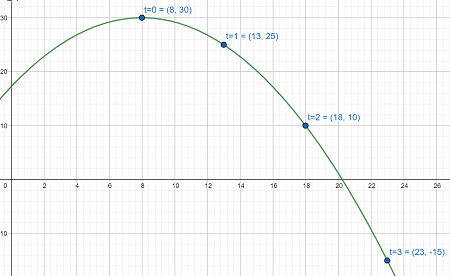# 2) a) x(t) = 5t + 8 and y(t) = 30 - 5t^2 give the table of values for 0 \leq t \leq 3. b) Give...

## Question:

2)

a) If {eq}x(t) = 5t + 8 {/eq} and {eq}y(t) = 30 - 5t^2 {/eq} give the table of values for {eq}0 \leq t \leq 3 {/eq}.

b) Give the graph for (a)

3) Find the derivatives for the following:

(a) {eq}f(x) = 4\, x \,e^x {/eq}

(b) {eq}f(x) =\frac{e^{2x}}{2x - 2} {/eq}

(c) {eq}y = (x^4 + 3x + 1)^3 {/eq}

## Parametric Equations:

Many times when graphing an equation, one variable, usually {eq}y {/eq} is written in terms of another variable, usually {eq}x, {/eq} and points can be found by picking values for the independent variable and calculating the corresponding values for the dependent variable. However, some curves are defined parametrically, meaning that both {eq}x {/eq} and {eq}y {/eq} are functions of some parameter {eq}t, {/eq} which can often be thought of as time. To graph a parametric curve, substitute values of {eq}t {/eq} into both {eq}x(t) \text{ and } y(t) {/eq} to plot points {eq}(x(t), y(t)) {/eq} and connect the points in the order of increasing {eq}t. {/eq}

2)

a) If {eq}x(t) = 5t + 8 {/eq} and {eq}y(t) = 30 - 5t^2 {/eq} give the table of values for {eq}0 \leq t \leq 3 {/eq}

To fill in the table of values, substitute values for {eq}t {/eq} into {eq}x(t) \text{ and } y(t). {/eq}

t x=5t+8 y=30-5t^2 (x,y)
0 8 30 (8,30)
1 13 25 (13, 25)
2 18 10 (18, 10)
3 23 -15 (23, -15)

b) Give the graph for (a)

Plotting the points found in the table and connecting, we have the curve below:3) Find the derivatives for the following:

(a) {eq}f(x) = 4xe^x {/eq}

Using the product rule, we have

{eq}f'(x) = (4x)(e^x) + (e^x)(4)\\ f'(x) = 4xe^x + 4e^x {/eq}

(b) {eq}f(x) =\frac{e^{2x}}{2x - 2} {/eq}

Using the quotient rule and the chain rule, we have

{eq}f'(x) = \dfrac{(2x-2)(e^{2x}\cdot 2) - (e^{2x})(2)}{(2x-2)^2}\\ f'(x) = \dfrac{e^{2x}(2(2x-2) - 2)}{(2x-2)^2}\\ f'(x) = \dfrac{e^{2x}(4x - 6)}{(2x-2)^2} {/eq}

(c) {eq}y = (x^4 + 3x + 1)^3 {/eq}

Using the chain rule, we have

{eq}y' = 3(x^4+3x+1)^2\cdot(4x^3+3)\\ y' = (12x^3 + 9)(x^4+3x+1)^2 {/eq}